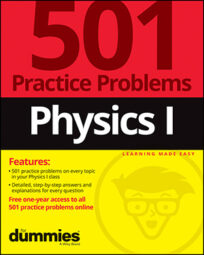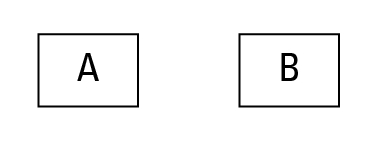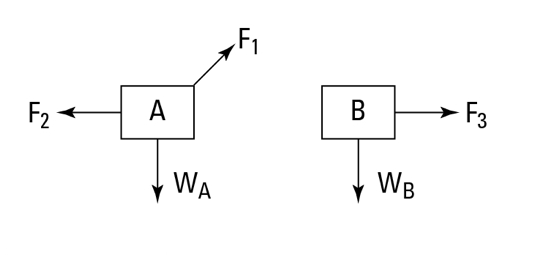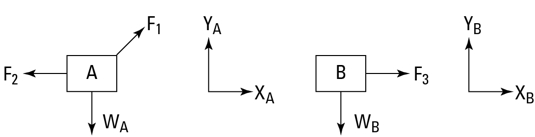##### Physics I: 501 Practice Problems For Dummies (+ Free Online Practice)Solving physics problems correctly is a lot easier when you have a couple tricks under your belt. In fact, you can greatly improve your odds of getting the right answer if you make sure that what you calculated is plausible in the real world. Another trick is to draw your own visual when one isn’t provided for you — no artistic ability required.

It also helps to have this handy reference for some of the most common unit prefixes and unit conversions you’re bound to encounter in your physics homework.

## How to check for physically reasonable answers in physics problems

Because physics describes reality, your solutions to any physics problems you tackle should be able to describe reality, too. You can avoid many mistakes by checking that your answers have the following properties:

• They have the right units. If a problem asks you to find a speed and you get 5 kilograms, you know you made a mistake somewhere. (Note that this check only works if you keep track of your units throughout the whole problem.)

• They’re the right size. If you calculate that the mass of a planet is 53 grams, that the speed of a soccer ball is 3 trillion meters per second, or that the temperature of ice is 350 degrees Celsius, start searching for the mistake.

• They point in the right direction. When you’re looking for a vector, sometimes you know roughly what direction it should point.

• They have the right sign. If you find that the density of a liquid is –1,200 kilograms per cubic meter, you made a sign error along the way.

## Solving force problems in physics by using free-body diagrams

In physics, force problems typically ask you to predict what will happen when you apply force to an object, and usually there’s no handy illustration to help you visualize what’s being described. Fortunately, you can create your own diagram so you can better picture what a question is asking you. Follow this seven-step method to solve force problems:

1. Draw each of the objects you’re interested in.

Here’s an example:2. Identify the forces acting on each object.

For each force acting on one of the objects from Step 1, draw an arrow that indicates the direction of the force, as shown in the following figure. Note that the tail of the arrow indicates which part of the object the force is acting on.3. Draw a free-body diagram for each object.

If you’re following this step-by-step guide, you’ve already drawn a free-body diagram. It’s listed separately because it’s the most important step!

4. Choose a coordinate system for each object.

Usually you draw the x-direction horizontally and the y-direction vertically, as shown in the following figure. However, when dealing with inclined planes, sometimes you want to choose your coordinate axes parallel and perpendicular to the plane.5. For each object, write down each component of Newton’s second law.

For angular motion problems, choose an axis of rotation and write down the angular version of Newton’s second law.

6. Include any constraints.

Sometimes you have more variables than equations at this point. Write down any other information you know. For example, if a car is driving on a flat road, you know that the vertical component of its acceleration is zero.

7. Solve the equations.

Now that you’ve used all your physics knowledge, all you have to do is the algebra.

## Physics reference charts for unit prefixes and unit conversion

When solving physics problems, you’ll often encounter unit prefixes that you need to know. You also need to be familiar with common unit symbols and their corresponding SI units. The following tables list some of the typical prefixes and symbols that you may see.

Commonly Used Unit Prefixes in Physics Problems
Unit Prefix Name Symbol Factor
tera- T 1012
giga- G 109
mega- M 106
kilo- k 103
centi- c 10–2
milli- m 10–3
micro- ì 10–6
nano- n 10–9
pico- p 10–12
Handy Unit Conversions for Physics Problems
Derived Units Symbol SI Units
Newton N kilogram meter per second squared
Joule J kilogram meter squared per second squared
Watt W kilogram meter squared per second cubed
Hertz Hz inverse second
Pascal P kilogram per meter per second squared
Degree Celsius °C kelvin